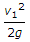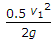# Mechanical Engineering - Hydraulics and Fluid Mechanics

### Exercise :: Hydraulics and Fluid Mechanics - Section 3

46.

The most economical section of a rectangular channel is one which has hydraulic mean depth or hydraulic radius equal to

 A. half the depth B. half the breadth C. twice the depth D. twice the breadth

Answer: Option A

Explanation:

No answer description available for this question. Let us discuss.

47.

The ratio of velocity of fluid in an undisturbed stream to the velocity of sound wave is known as Mach number.

 A. Yes B. No

Answer: Option A

Explanation:

No answer description available for this question. Let us discuss.

48.

The length AB of a pipe ABC in which the liquid is flowing has diameter (d1) and is suddenly contracted to diameter (d2) at B which is constant for the length BC. The loss of head due to sudden contraction, assuming coefficient of contraction as 0.62, is

 A.B.C.D.Answer: Option D

Explanation:

No answer description available for this question. Let us discuss.

49.

All the gases are considered to have compressible flow and all the liquids are considered to have incompressible flow.

 A. Correct B. Incorrect

Answer: Option A

Explanation:

No answer description available for this question. Let us discuss.

50.

When a cylindrical vessel containing liquid is revolved, the surface of the liquid takes the shape of

 A. a triangle B. a paraboloid C. an ellipse D. none of these

Answer: Option B

Explanation:

No answer description available for this question. Let us discuss.

#### Current Affairs 2021

Interview Questions and Answers